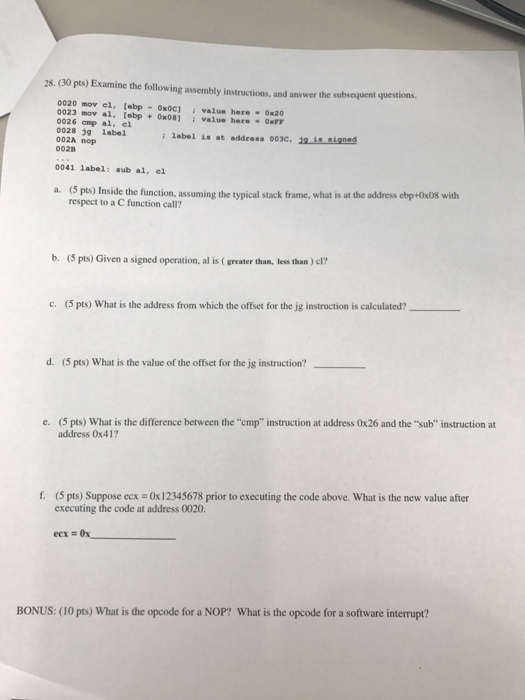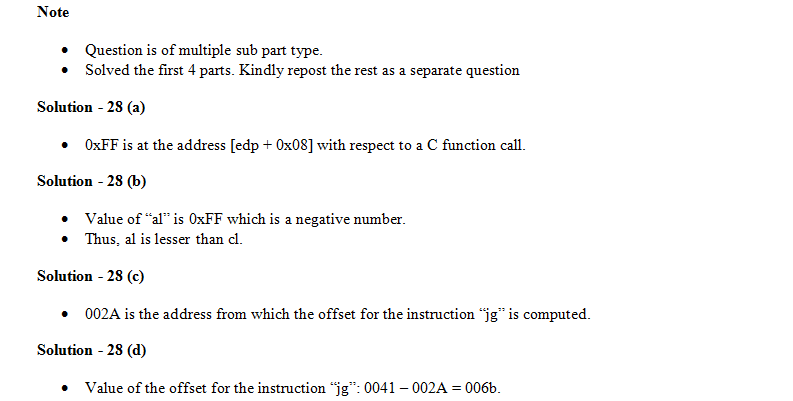# Question & Answer: Examine the following assembly instructions, and answer the subsequent questions. a. Inside the f…..Help please

Examine the following assembly instructions, and answer the subsequent questions. a. Inside the function, assuming the typical stack frame, what is at the address ebp + 0 times 08 with respect to a C function call? b. Given a signed operation, al is (greater than, less than) cl? c. What is the address from which the offset for the jg instruction is calculated? _____ d. What is the value of the offset for the jg instruction? e. What is the difference between the “cmp” instruction at address 0 times 26 and the “sub” instruction at address 0 times 41? f. Suppose ecx = 0 times 12345678 prior to executing the code above. What is the new value after executing the code at address 0020. exc = 0x _____ BONUS: What is the opcode for a NOP? What is the opcode for a software interrupt?